New Foundations Explorer < Previous   Next > Nearby theorems Mirrors  >  Home  >  NFE Home  >  Th. List  >  wi Unicode version

Syntax Definition wi 4
 Description: If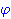and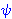are wff's, so is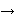or "implies." Part of the recursive definition of a wff. The resulting wff is (interpreted as) false whenis true andis false; it is true otherwise. Think of the truth table for an OR gate with inputconnected through an inverter. After we define the axioms of propositional calculus (ax-1 5, ax-2 6, ax-3 7, and ax-mp 8), the biconditional (df-bi 177), the constant true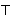(df-tru 1319), and the constant false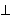(df-fal 1320), we will be able to prove these truth table values: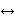(truimtru 1344),(truimfal 1345),(falimtru 1346), and(falimfal 1347). These have straightforward meanings, for example,just means "the value ofis". The left-hand wff is called the antecedent, and the right-hand wff is called the consequent. In the case of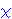, the middlemay be informally called either an antecedent or part of the consequent depending on context. Contrast with(df-bi 177),(df-an 360), and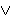(df-or 359). This is called "material implication" and the arrow is usually read as "implies." However, material implication is not identical to the meaning of "implies" in natural language. For example, the word "implies" may suggest a causal relationship in natural language. Material implication does not require any causal relationship. Also, note that in material implication, if the consequent is true then the wff is always true (even if the antecedent is false). Thus, if "implies" means material implication, it is true that "if the moon is made of green cheese that implies that 5=5" (because 5=5). Similarly, if the antecedent is false, the wff is always true. Thus, it is true that, "if the moon made of green cheese that implies that 5=7" (because the moon is not actually made of green cheese). A contradiction implies anything (pm2.21i 123). In short, material implication has a very specific technical definition, and misunderstandings of it are sometimes called "paradoxes of logical implication."
Hypotheses
Ref Expression
wphwpsAssertion
Ref Expression
wiThis syntax is primitive. The first axiom using it is ax-1 5.

 Colors of variables: wff setvar class
 Copyright terms: Public domain W3C validator